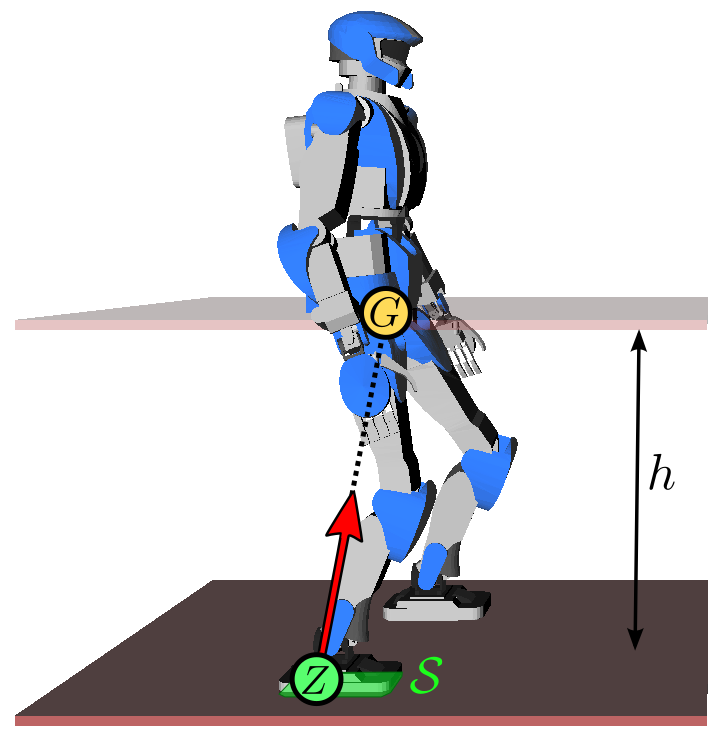# Linear inverted pendulum model

The linear inverted pendulum model focuses on the translational components of a legged robot's dynamics.

## Derivation

Both fixed and mobile robots are usually modeled as rigid bodies connected by actuated joints. The equation of motion for such a system are:

\begin{equation*} \bfM(\bfq) \qdd + \qd^\top \bfC(\bfq) \qd = \bfS^\top \bftau + \bftau_g(\bfq) + \sum_{i=1}^N \bfJ_{C_i}^\top \bff_i, \end{equation*}

where $$\bfq$$ is the vector of actuated and unactuated coordinates. Actuated coordinates correspond to joint angles directly controlled by motors. Unactuated coordinates correspond to the six degrees of freedom for the position and orientation of the floating base (a frame attached to any of the robot's bodies) with respect to the inertial fame. The vector $$\bfq$$ is typically high-dimensional.

### Centroidal dynamics

The first working assumption to simplify this model is (Assumption 1) that the robot has enough joint torques to realize the actuated part of the equation, and focus on the Newton-Euler equations that correspond to the six unactuated coordinates:

\begin{equation*} \begin{bmatrix} m \bfpdd_G \\ \dot{\bfL}_G \end{bmatrix} = \begin{bmatrix} \bff \\ \bftau_G \end{bmatrix} + \begin{bmatrix} m \bfg \\ \boldsymbol{0} \end{bmatrix} \end{equation*}

where on the left-hand side $$\bfp_G$$ is the position of the center of mass (CoM) and $$\bfL_G$$ is the net angular momentum around the CoM, while on the right-hand side $$\bff$$ is the resultant of contact forces, $$\bftau_G$$ is the moment of contact forces around the CoM, $$m$$ is the robot mass and $$\bfg$$ is the gravity vector. This model is called centroidal dynamics.

### Linearized dynamics

Angular momentum or height variations make centroidal dynamics nonlinear. This means for instance that, to generate a trajectory for this system, one needs to solve a nonlinear optimization. An alternative to linearize this system is to make two assumptions:

• Assumption 2: there is no angular momentum around the center of mass $$(\dot{\bfL}_G=\boldsymbol{0})$$. This is why the Honda P2 walks with locked arms.
• Assumption 3: the center of mass keeps a constant height. This is why the Honda P2 walks with bent knees.

These two assumptions are used to derive linearized dynamics as follows. First, let us consider the zero-tilting moment point (ZMP) of the contact wrench. It is a point $$Z$$ where the moment of contact forces is vertical:

\begin{equation*} \bfe_Z \times \bftau_Z = \boldsymbol{0} \end{equation*}

with $$\bfe_Z$$ the unit upward vertical vector of the inertial frame. This quantity defines an axis in general: to make $$Z$$ a unique point, let us take it on the ground with $$z_Z = z_G - h$$, where $$h$$ is the constant height of the CoM. The moment $$\bftau_Z$$ of the contact wrench at this ZMP is related to the moment at the CoM by:

\begin{equation*} \bftau_Z = \bftau_G + (\bfp_G - \bfp_Z) \times \bff \end{equation*}

Since $$\bftau_G = \boldsymbol{0}$$ (Assumption 2), we have:

\begin{equation*} \bfe_Z \times ((\bfp_G - \bfp_Z) \times \bff) = \boldsymbol{0} \end{equation*}

Applying the vector triple product formula, we get:

\begin{equation*} f_z (\bfp_G - \bfp_Z) - h \bff = \boldsymbol{0} \end{equation*}

From Newton's equation, $$\bff = m (\bfpdd_G - \bfg)$$ and we can rewrite the equation above as:

\begin{equation*} h (\bfpdd_G - \bfg) = (\zdd_G + g) (\bfp_G - \bfp_Z) \end{equation*}

Since $$\zdd_G = 0$$ (Assumption 3), this equation is a trivial identity in the vertical direction while its horizontal coordinates are:

\begin{equation*} \bfpdd_G = \omega^2 (\bfp_G - \bfp_Z) \end{equation*}

where $$\omega^2 = g / h$$, $$g$$ is the gravity constant and $$h = z_G - z_Z$$ is the constant height of the center of mass. The constant $$\omega$$ is called natural frequency of the linear inverted pendulum.In this model, the robot can be seen as a point-mass concentrated at $$G$$ resting on a mass-less leg in contact with the ground at $$Z$$. Intuitively, the ZMP is the point where the robot applies its weight. As a consequence, this point needs to lie inside the contact surface $$\cal S$$.

## To go further

The linear inverted pendulum mode (not model) was introduced in Kajita et al. (2001) for walking pattern generation. The rationale for calling it a mode of motion is that it relies solely on planar linear momentum, which is one among many ways to affect the acceleration of the center of mass and thus locomote (others include height variations and angular momentum variations that separate the ZMP and centroidal moment pivot).

Pages of this website are under the CC-BY 4.0 license.